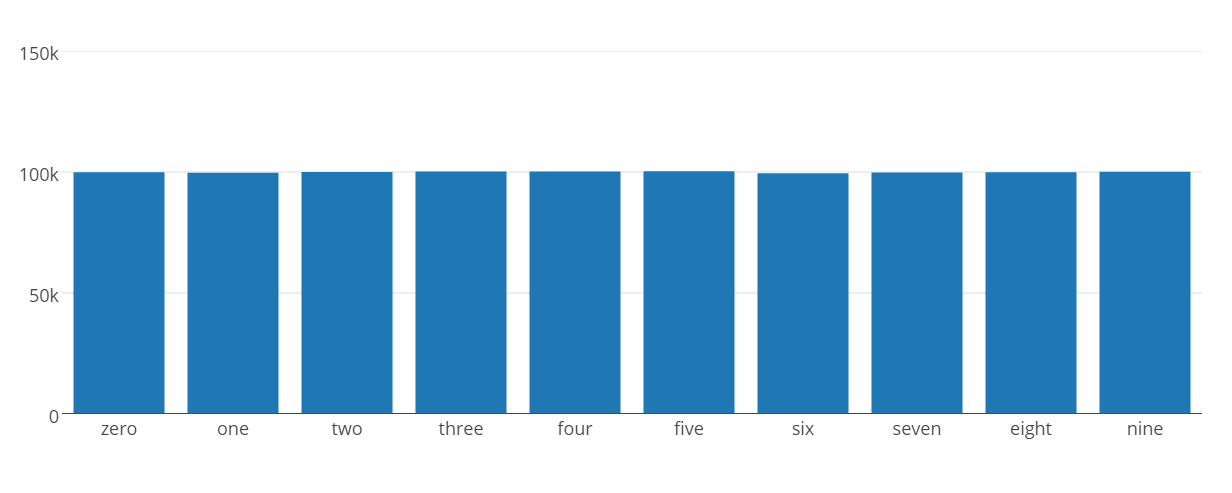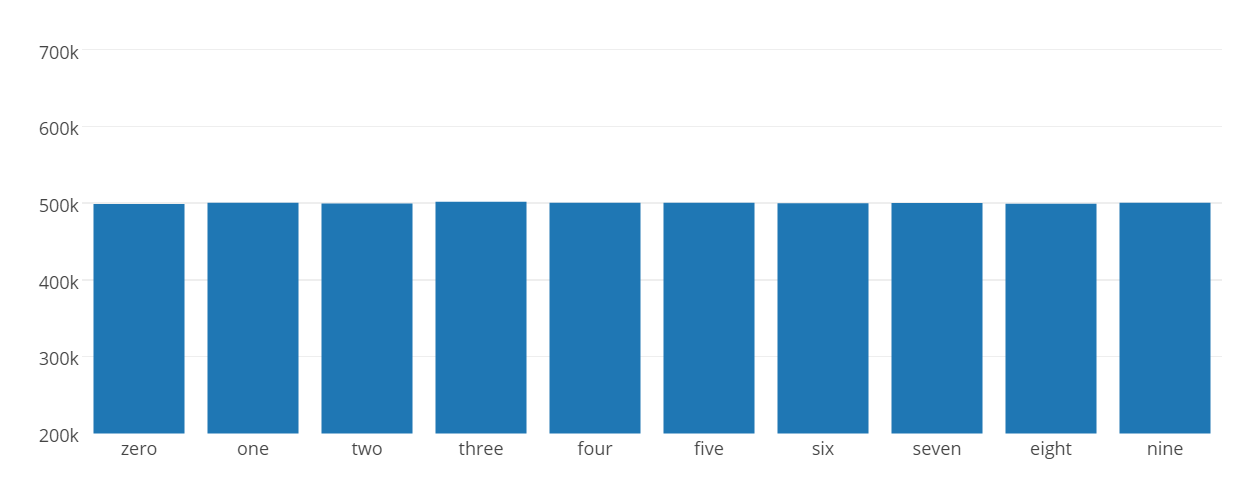# 【Overview】

I checked the frequency of numbers that appear in pi. I also checked the number of Napiers. The target is after the decimal point.

# [Preparation]

-Obtain the API key from Plotly to use the Python library Plotly. (Not required if you do not draw a graph) ・ Pi and Number of Napiers Copy and paste the numbers after the decimal point into the text from /e.5mil). (Note that the number of Napiers is filled with 0 because the first line has a different digit from the other lines.)

# [Folder structure]

|---scripts | --- pi.py (Check the frequency of pi numbers) | --- pi_graph.py (Create a bar graph of pi) | --- pi.txt (Pi after the decimal point) | --- e.py (Check the frequency of Napier numbers) | --- e_graph.py (Create bar graph of Napier number) | --- e.txt (number of napiers after the decimal point)

# [Program and results]

To display the graph https://plot.ly/~ユーザ名/0/#plot You can see it by accessing.

#### `pi.py`

``````
# -*- coding: utf-8 -*-

zero = 0
one = 0
two = 0
three = 0
four = 0
five = 0
six = 0
seven = 0
eight = 0
nine = 0

for line in open('pi.txt', 'r'):
for i in xrange(100):
if(line[i] == '0'):
zero += 1
elif(line[i] == '1'):
one += 1
elif(line[i] == '2'):
two += 1
elif(line[i] == '3'):
three += 1
elif(line[i] == '4'):
four += 1
elif(line[i] == '5'):
five += 1
elif(line[i] == '6'):
six += 1
elif(line[i] == '7'):
seven += 1
elif(line[i] == '8'):
eight += 1
elif(line[i] == '9'):
nine += 1
print zero    #99959
print one     #99758
print two     #100026
print three   #100229
print four    #100230
print five    #100359
print six     #99548
print seven   #99800
print eight   #99985
print nine    #100106
``````

#### `e.py`

``````
# -*- coding: utf-8 -*-

zero = -3 #I've filled the first line of text with three zeros, so I'll reduce it.
one = 0
two = 0
three = 0
four = 0
five = 0
six = 0
seven = 0
eight = 0
nine = 0

for line in open('e.txt', 'r'):
for i in xrange(60):
if(line[i] == '0'):
zero += 1
elif(line[i] == '1'):
one += 1
elif(line[i] == '2'):
two += 1
elif(line[i] == '3'):
three += 1
elif(line[i] == '4'):
four += 1
elif(line[i] == '5'):
five += 1
elif(line[i] == '6'):
six += 1
elif(line[i] == '7'):
seven += 1
elif(line[i] == '8'):
eight += 1
elif(line[i] == '9'):
nine += 1
print zero    #498642
print one     #500511
print two     #499302
print three   #501715
print four    #500420
print five    #500489
print six     #499875
print seven   #500015
print eight   #499078
print nine    #500290
``````

#### `pi_graph.py`

``````
# -*- coding: utf-8 -*-

import plotly
import plotly.plotly as py
import plotly.graph_objs as go
plotly.tools.set_credentials_file(username='User name', api_key='API key')

data = [go.Bar(
x=['zero', 'one', 'two', 'three', 'four', 'five', 'six', 'seven', 'eight', 'nine'],
y=[99959, 99758, 100026, 100229, 100230, 100359, 99548, 99800, 99985, 100106]
)]

py.iplot(data, filename='basic-bar')
``````#### `e_graph.py`

``````
# -*- coding: utf-8 -*-

import plotly
import plotly.plotly as py
import plotly.graph_objs as go
plotly.tools.set_credentials_file(username='User name', api_key='API key')

data = [go.Bar(
x=['zero', 'one', 'two', 'three', 'four', 'five', 'six', 'seven', 'eight', 'nine'],
y=[498642, 500511, 499302, 501715, 500420, 500489, 499875, 500015, 499078, 500290]
)]

py.iplot(data, filename='basic-bar')
``````# [Discussion]

It seems that both the pi and the number of Napiers appear at the same frequency ... Is there a mathematical theorem that already appears at the same frequency? (If you know it, please let me know in the comments)

# [Reference site]

https://plot.ly/python/bar-charts/